Entropy & its Physical Significance

# Entropy & its Physical Significance - Notes | Study Physical Chemistry - Chemistry

 1 Crore+ students have signed up on EduRev. Have you?

Entropy:  Let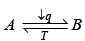then entropy change for a finite change of state of a system at constant temperature is given by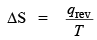Unit of entropy is JK–1.
Entropy is an extensive property. Its value depends upon the amount of the substance involved.
Entropy change for an ideal gas: According to first law, we have dqrev = dV + PdV
Dividing T; we have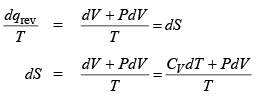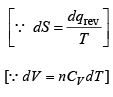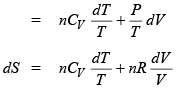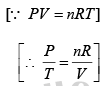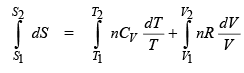or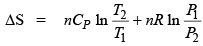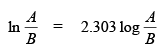This is the expression when both the volume and temperature or pressure and temperature of an ideal gas are changed.
For an isothermal process, the change is given by the relation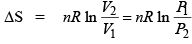∵ T2 = T1

and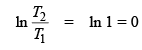For the change of temperature at constant volume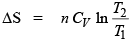For the change of temperature at constant pressure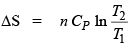Entropy change in a few typical cases.

Entropy change in a reversible phase transformat ion.

Since the reversible phase transformation takes place at constant equilibrium temperature then entropy.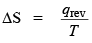where qrev is the heat invo lved in the phase transformat ion.
Examples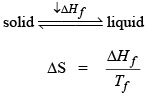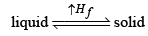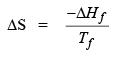Entropy change in an irreversible phase transition.
A → B
then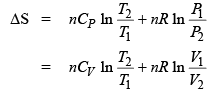Problem.  The phase transformation of one mole of liquid water at –10°C (T1) to solid water –10°C can be calculated following the paths given below: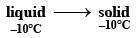(i)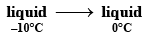ΔCPl = 75.312 JK-1 mol-1
(ii)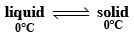ΔHf = 6088.2 J mol-1

(iii)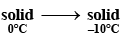CPs = 36.401 JK-1 mol-1

Then changes of entropy can be calculated
Sol.
(i)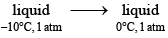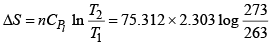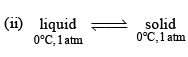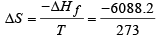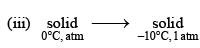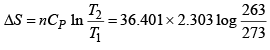ΔS = -1.358 JK mol -1

then, the change of the entropy of the process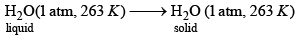is given by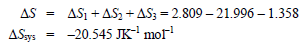Entropy change when two solid at different temperatures are brought together: Let one at higher temperature Th and other at lower temperature Tc. When two bodies are brought together then
Heat lost = heat gain

(by conservat ion law)

i.e. Heat lost by hot body = Heat gained by cold body
Cp(Th – T) = Cp (T – Tc)
or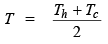Entropy changes of two bodies are bodies are: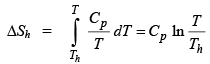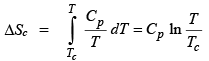The total change of entropy is   ΔStotal = ΔSh + ΔS

Problem. 5.0 gm ice at 273 K is added to 30 gm of water at 323 K in a thermally insulated container.
What is the final temperature?

ΔHfus of ice at 273 K = 334.72 J gm-1
Cp of water = 4.184 JK-1 gm-1

ΔHvap of water at 373 K = 2.259 J gm-1

Sol. Heat require to convert 5 gm of ice at 273 K to 5 gm water at 273 K
= (5 g) × (334.72 J gm-1) = 1673.6 J

Final temperature after mixing
Heat gained by ice = Heat lost by hot water

(5 gm) (334.72 J gm–1) + (5 g) (4.184 J gm–1 K–1) (T – 273 K)
= (30 g) (4.184 JK–1 gm–1) (323 K – T)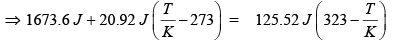Solving for T, we get
T = 304.43 K

Entropy of mixing: Entropy of mixing is defined as the difference between the entropy of the mixture of gas and the sum of the entropy of the separate gases, each at a pressure P.
For a mole of an ideal gas,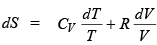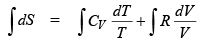S = CV ln T + R ln V + S0

where S0 is the integration constant.
We know that,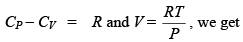, we get S = CP ln T – R ln P + R ln R + S0

= CP ln T – R ln P + S0’ …(1)

Where         S0 = (R ln R + S0) is another constant.
If n1, n2, …. etc. are the number of moles of the various gases present in the mixture and p1, p2, … etc. are their partial pressures, then the entropy of the mixture is given by

S = n(Cp ln T –R ln P1 + S0’) + n2(Cp ln T – R ln P2 + S0’) + ……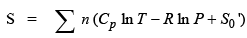...............(2)

The partial pressure (p) of an ideal gas is given by P = xp where x is the mole fract ion and P is the total pressure.
Putting this value we get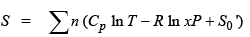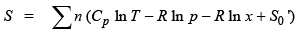...............(3)

Equation (3) gives the entropy of a mixture of ideal gases. “Entropy of mixing is difference between the entropy of the mixture of gases and the sum of the entropies of the separate gases”
Thus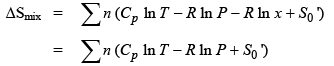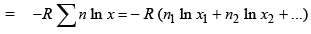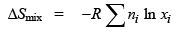where ni and nx are number of moles and mole fraction of each constituent of the mixture.
If n is the total number of moles then
n = n1 + n2 ……..
then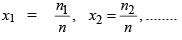then molar entropy of gases are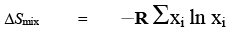Problem.  Calculate the entropy of mixing of one mole of nitrogen gas and three moles of hydrogen gas, assuming that no chemical reaction occurs. i.e. mixing is ideal.
Sol.

n1 = 1,  n2 = 3

The mole fract ion are: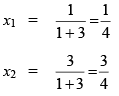form equation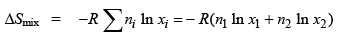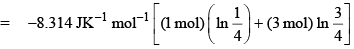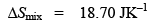Physical Significance of Entropy:

(1)  Entropy as a measure of the disorder of the system: Spontaneous process are accompanied by increase in entropy as well as increase in the disorder of the system.
Thus, entropy is a measure of the disorder of a system.

(2)  Entropy as a measure of probability: All spontaneous process lead to increase in entropy and also to increase in disorder. It appears that there is a close relation between ‘entropy S and the probability (W).

This relationship was expressed by Boltzmann as
S = k ln (Probabilit y) = kln (W)

S = k ln W

where k is Boltzmann constant
S = k ln W = k ln (arrangement)NA
= k NA ln (arrangement)
S = R ln (arrangement)

The document Entropy & its Physical Significance - Notes | Study Physical Chemistry - Chemistry is a part of the Chemistry Course Physical Chemistry.
All you need of Chemistry at this link: Chemistry

## Physical Chemistry

85 videos|131 docs|67 tests
 Use Code STAYHOME200 and get INR 200 additional OFF

## Physical Chemistry

85 videos|131 docs|67 tests

Track your progress, build streaks, highlight & save important lessons and more!

,

,

,

,

,

,

,

,

,

,

,

,

,

,

,

,

,

,

,

,

,

;